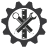SuperTeacherTools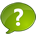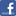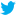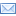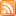O'Brien Multiplication Speed Match Review Game

This game has been played times
The best time ever was at seconds.
The best time today is at seconds.
How To Play:
Drag the squares in the gray area and drop them in the matching squares in the blue area below.
If you drop a square in the correct spot, it will disappear.
If you make a mistake, the square will return to its original spot.
Good luck!

Let's Play Speed Match!

8, 16, 24, 32, 40, 48, 56
9
36
1600
zero
36
7, 14, 21, 28, 35, 42, 49
10, 20, 30, 40, 50, 60, 70
28
An even number
Either 5 or 0
24
8+8+8+8+8+8 or 6 x 8
56
a picture showing a multiplication problem
19
2+2+2+2+2+2+2 or 7+7
24
10 x20, 1 x20 or 4x 5
35
3, 6, 9, 12, 15, 18, 21
49
12, 24, 36, 48, 60, 72, 84
If it rained 7 inches every day for 7 days, how many inches did it rain all together?
If you multiply an even number by an even number what will your product always be?
Michael wanted to borrow video games from his friends. He wanted 27 games all together. If each friend gave him 3 games each, how many friends did he have to borrow from?
What is the repeated addition that would show 2 x 7
What are the multiples of 10
Draw an array that shows 4 x 6
5 x 7
What are the multiples of 3
20 x 80
There are six bookshelves, each bookshelf has eight books. How would you write the repeated addition AND the multiplication equation for this problem
7 x 8
What is an array
What are the multiples of 8
What are the multiples of 12
4 x 6
If there are 3 friends and they each have 12 peaches, how many peaches do they have all together?
A teacher was counting her class by 5's, when she finished counting she ended on 140, how many students did she have in her class?
2 x 9
What are the multiples of 7
If I had an array that was 8 x 3, what would the product be?
If one of your factors is zero, what will your product be?
If you multiply by 5, what two numbers will the product always end in?
How could I break down a 6 x 7 array into smaller arrays?
If I had an array that had a product of 20, what could the dimensions be?